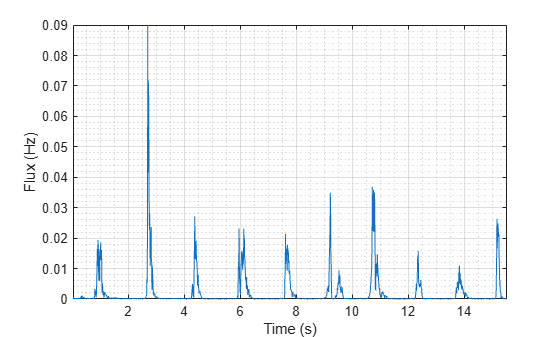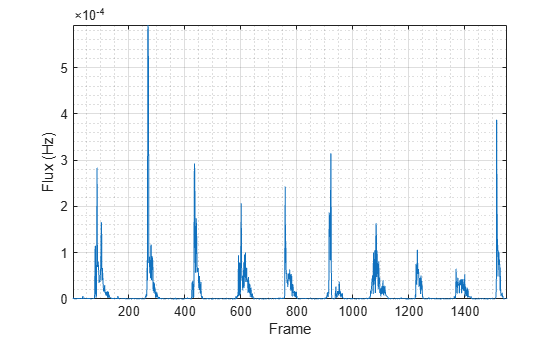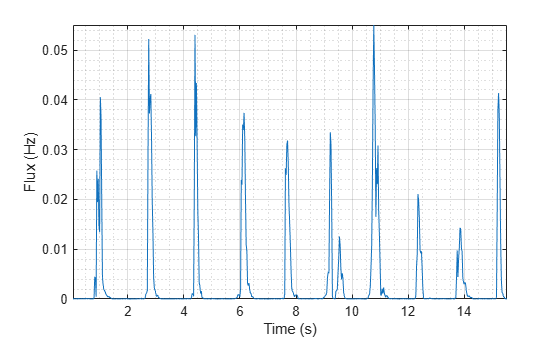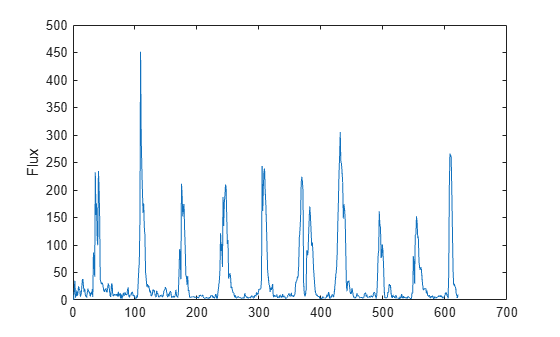# spectralFlux

Spectral flux for audio signals and auditory spectrograms

## Syntax

``flux = spectralFlux(x,f)``
``flux = spectralFlux(x,f,initialCondition)``
``flux = spectralFlux(___,Name,Value)``
``[flux,finalCondition] = spectralFlux(___)``

## Description

example

````flux = spectralFlux(x,f)` returns the spectral flux of the signal, `x`, over time. Spectral flux is a measure of the variability of the spectrum over time. How the function interprets `x` depends on the shape of `f`.```

example

````flux = spectralFlux(x,f,initialCondition)` specifies the previous spectral state. This syntax is supported only for frequency-domain inputs.```

example

````flux = spectralFlux(___,Name,Value)` specifies options using one or more `Name,Value` pair arguments.For example, `flux = spectralFlux(x,f,'NormType',1)` calculates spectral flux using norm type 1.```

example

````[flux,finalCondition] = spectralFlux(___)` also returns the final spectral state.```

## Examples

collapse all

Read in an audio file, calculate the flux using default parameters, and then plot the results.

```[audioIn,fs] = audioread('Counting-16-44p1-mono-15secs.wav'); flux = spectralFlux(audioIn,fs); t = linspace(0,size(audioIn,1)/fs,size(flux,1)); plot(t,flux) xlabel('Time (s)') ylabel('Flux')```Read in an audio file and then calculate the mel spectrogram using the `melSpectrogram` function. Calculate the flux of the mel spectrogram over time. Plot the results.

```[audioIn,fs] = audioread('Counting-16-44p1-mono-15secs.wav'); [s,cf,t] = melSpectrogram(audioIn,fs); flux = spectralFlux(s,cf); plot(t,flux) xlabel('Time (s)') ylabel('Flux')````[audioIn,fs] = audioread('Counting-16-44p1-mono-15secs.wav');`

Calculate the flux of the power spectrum over time. Calculate the flux for 50 ms Hamming windows of data with 25 ms overlap. Use the range from 62.5 Hz to `fs`/2 for the flux calculation. Plot the results.

```flux = spectralFlux(audioIn,fs, ... 'Window',hamming(round(0.05*fs)), ... 'OverlapLength',round(0.025*fs), ... 'Range',[62.5,fs/2]); t = linspace(0,size(audioIn,1)/fs,size(flux,1)); plot(t,flux) xlabel('Time (s)') ylabel('Flux')```Spectral flux measures the change in consecutive spectra. To calculate spectral flux of streaming audio, you can pass the state in and out of the function.

Create a `dsp.AudioFileReader` object to read in audio data frame-by-frame. Create a `dsp.AsyncBuffer` object to buffer the audio input into overlapped frames. Create a second `dsp.AsyncBuffer` object to log the spectral flux calculation.

```fileReader = dsp.AudioFileReader('Counting-16-44p1-mono-15secs.wav'); inputBuffer = dsp.AsyncBuffer; logger = dsp.AsyncBuffer;```

In an audio stream loop:

2. Write the audio data to the input buffer.

3. If a hop of data is available from the buffer, read a frame of data with overlap.

4. Calculate the one-sided magnitude short time Fourier transform.

5. Calculate the spectral flux.

6. Log the spectral flux for later plotting.

```fs = fileReader.SampleRate; samplesPerFrame = round(fs*0.05); samplesOverlap = round(fs*0.025); samplesPerHop = samplesPerFrame - samplesOverlap; win = hamming(samplesPerFrame,'periodic'); Sprev = []; while ~isDone(fileReader) audioIn = fileReader(); write(inputBuffer,audioIn); while inputBuffer.NumUnreadSamples >= samplesPerHop audioBuffered = read(inputBuffer,samplesPerFrame,samplesOverlap); [S,f] = stft(audioBuffered,fs,"Window",win,"OverlapLength",samplesOverlap,"FrequencyRange","onesided"); [flux,Sprev] = spectralFlux(abs(S),f,Sprev); write(logger,flux); end end release(fileReader)```

Plot the logged data.

```plot(read(logger)) ylabel('Flux')```## Input Arguments

collapse all

Input signal, specified as a vector, matrix, or 3-D array. How the function interprets `x` depends on the shape of `f`.

Data Types: `single` | `double`

Sample rate or frequency vector in Hz, specified as a scalar or vector, respectively. How the function interprets `x` depends on the shape of `f`:

• If `f` is a scalar, `x` is interpreted as a time-domain signal, and `f` is interpreted as the sample rate. In this case, `x` must be a real vector or matrix. If `x` is specified as a matrix, the columns are interpreted as individual channels.

• If `f` is a vector, `x` is interpreted as a frequency-domain signal, and `f` is interpreted as the frequencies, in Hz, corresponding to the rows of `x`. In this case, `x` must be a real L-by-M-by-N array, where L is the number of spectral values at given frequencies of `f`, M is the number of individual spectra, and N is the number of channels.

Data Types: `single` | `double`

Previous spectral state, specified as an L-by-N matrix, where:

• L is the number of bins in the one-sided spectral representation, equal to `numel(f)`.

• N is the number of channels of audio data, equal to `size(x,3)`.

If `initialCondition` is unspecified, or specified as an empty, `spectralFlux` considers the first spectrum as repeating. That is, the first `flux` output is zero.

#### Dependencies

This input argument is only valid if the input, `x`, is a frequency-domain representation of audio. The `spectralFlux` function interprets the domain of the input `x` based on the size of `f`.

Data Types: `single` | `double`

### Name-Value Arguments

Specify optional comma-separated pairs of `Name,Value` arguments. `Name` is the argument name and `Value` is the corresponding value. `Name` must appear inside quotes. You can specify several name and value pair arguments in any order as `Name1,Value1,...,NameN,ValueN`.

Example: `'Window',hamming(256)`

Norm type used to calculate flux, specified as the comma-separated pair consisting of `'NormType'` and `2` or `1`.

Data Types: `single` | `double`

Note

The following name-value pair arguments apply if `x` is a time-domain signal. If `x` is a frequency-domain signal, the following name-value pair arguments are ignored.

Window applied in the time domain, specified as the comma-separated pair consisting of `'Window'` and a real vector. The number of elements in the vector must be in the range [1, `size(x,1)`]. The number of elements in the vector must also be greater than `OverlapLength`.

Data Types: `single` | `double`

Number of samples overlapped between adjacent windows, specified as the comma-separated pair consisting of `'OverlapLength'` and an integer in the range [0, `size(Window,1)`).

Data Types: `single` | `double`

Number of bins used to calculate the DFT of windowed input samples, specified as the comma-separated pair consisting of `'FFTLength'` and a positive scalar integer. If unspecified, `FFTLength` defaults to the number of elements in the `Window`.

Data Types: `single` | `double`

Frequency range in Hz, specified as the comma-separated pair consisting of `'Range'` and a two-element row vector of increasing real values in the range [0, `f`/2].

Data Types: `single` | `double`

Spectrum type, specified as the comma-separated pair consisting of `'SpectrumType'` and `'power'` or `'magnitude'`:

• `'power'` –– The spectral flux is calculated for the one-sided power spectrum.

• `'magnitude'` –– The spectral flux is calculated for the one-sided magnitude spectrum.

Data Types: `char` | `string`

## Output Arguments

collapse all

Spectral flux in Hz, returned as a scalar, vector, or matrix. Each row of `flux` corresponds to the spectral flux of a window of `x`. Each column of `flux` corresponds to an independent channel.

Final spectral state, returned as an L-by-N matrix, where:

• L is the number of bins in the one-sided spectral representation, equal to `numel(f)`.

• N is the number of channels of audio data, equal to `size(x,3)`.

#### Dependencies

This output argument is only valid if the input, `x`, is a frequency-domain representation of audio. The `spectralFlux` function interprets the domain of the input `x` based on the size of `f`.

## Algorithms

The spectral flux is calculated as described in :

`$\text{flux(}t\text{)}={\left(\sum _{k={b}_{1}}^{{b}_{2}}{|{s}_{k}\left(t\right)-{s}_{k}\left(t-1\right)|}^{P}\right)}^{1}{P}}$`

where

• sk is the spectral value at bin k.

• b1 and b2 are the band edges, in bins, over which to calculate the spectral flux.

• P is the norm type. You can specify the norm type using `NormType`.

 Scheirer, E., and M. Slaney. "Construction and Evaluation of a Robust Multifeature Speech/Music Discriminator." IEEE International Conference on Acoustics, Speech, and Signal Processing. Volume 2, 1997, pp. 1221–1224.

## Support평가판 신청